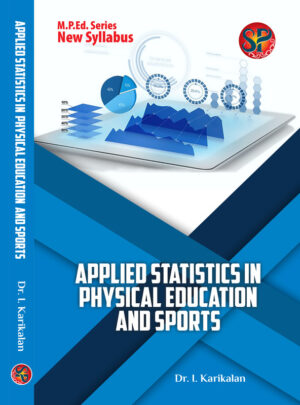# Applied Statistics in Physical Education and Sports (M.P.Ed. New Syllabus)

UNIT I – Meaning and Definition of Statistics. Function, need and importance of Statistics. Types of Statistics. Meaning of the terms: Population, Sample, Data, Kinds of data. Variables: Discrete and Continuous. Parametric and non-parametric statistics ||  UNIT II – Meaning, uses and construction of frequency table. Meaning, Purpose, Calculation and advantages of Measures of central tendency -Mean, median and mode. || UNIT III- Meaning, Purpose, Calculation and advantages of Measures of Variability -Range, Quartile Deviation, Mean Deviation, Standard Deviation and Probable Error. Meaning, Purpose, Calculation and advantages of scoring scales: Sigma scale, Z Scale, Hull scale and T scale. || UNIT IV – Normal Curve: Meaning of probability – Principles of normal curve – Properties of normal curve. Divergence form normality – Skewness and Kurtosis. Graphical Representation in Statistics: Line Diagram, Pie diagram, Bar diagram, Histogram, Frequency Polygon, Ogive Curve. || UNIT V- Tests of significance: Independent “t” test, Dependent “t’ test – chi – square test, level of confidence and interpretation of data. Meaning of correlation – co- efficient of correlation – calculation of co-efficient of correlation by the product moment method and rank difference method. Concept of ANOVA and ANCOVA.

400.00

Author Dr. I. Karikalan Hardcover First 2020

## Reviews

There are no reviews yet.